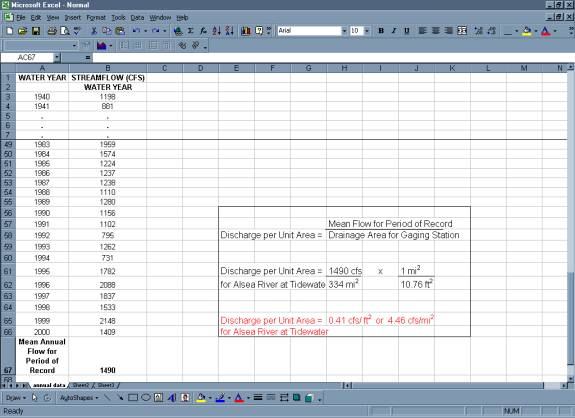# Analysis Techniques: Annual Analysis Example

## Step 1:Calculating Mean Annual Flow

• You can use the average function in Excel to obtain the average streamflow for each water year in the period of record.  The averaging will involve 365 or 366 daily values in each water year (366 days accounts for leap year). See the Data Manipulation section for a more detailed explanation of how to obtain data and to calculate mean annual flow.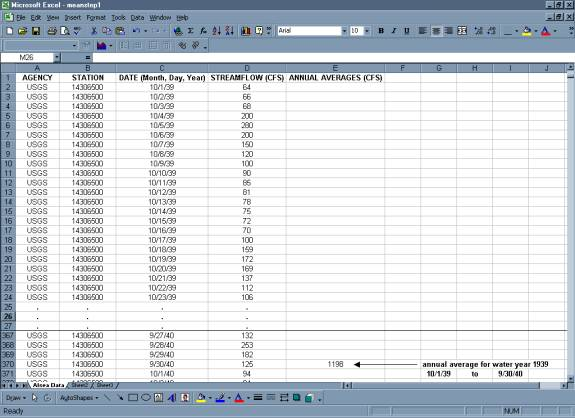• Copy your mean flow for each year into a new table.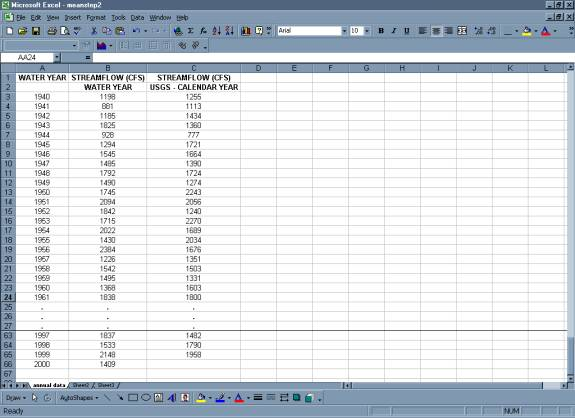## Step 2:  Calculating the Mean Annual Flow for Period of Record

• Use the average function to calculate the mean annual flow for the period of record. For this example, the period of record is 10 years.
• Column C in the table below contains the mean annual flow for "calendar years". It is interesting to compare how the value changes when the mean is calculated for "calendar years" instead of "water years".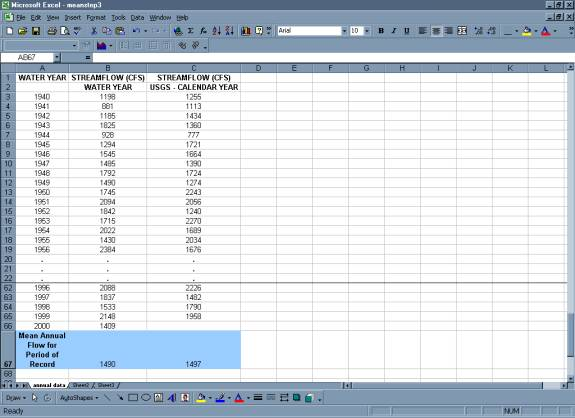## Step 3:  Ranked Annual Flow

• Use the sort option (under the Data menu) to sort mean annual flows in descending order.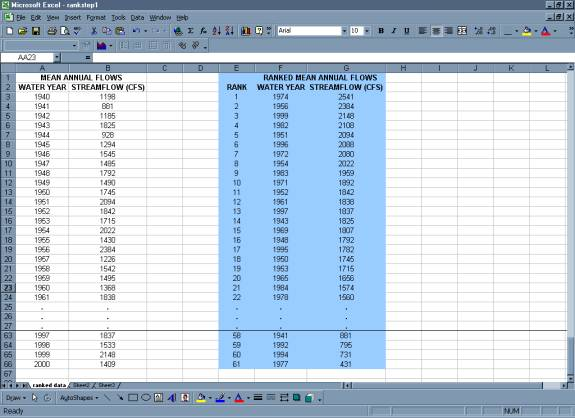• Plot ranked data.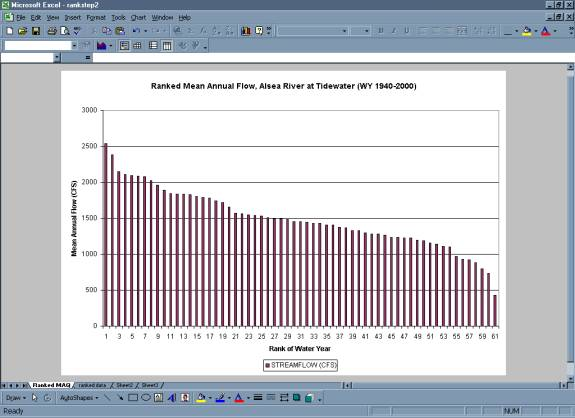• It may be helpful to plot on the chart the mean annual flow and one standard deviaton of the mean.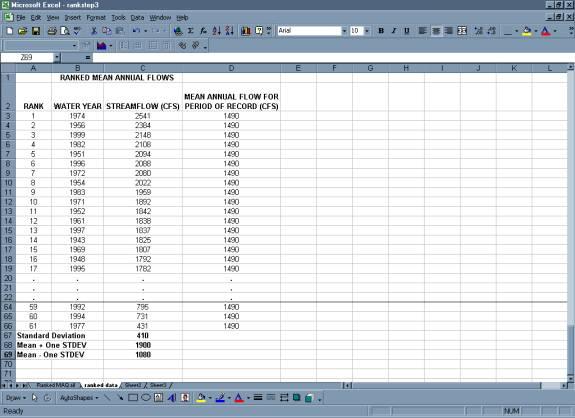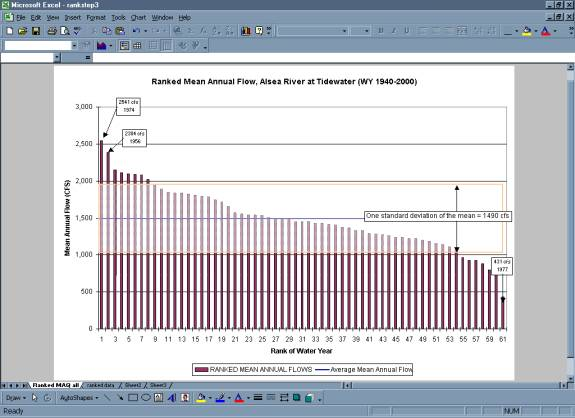## Step 4:  Pattern Analysis

### Step 4a:  Variation of Mean Annual Flow around Mean Flow for Period of Record

• Plot mean annual flow vs. water year.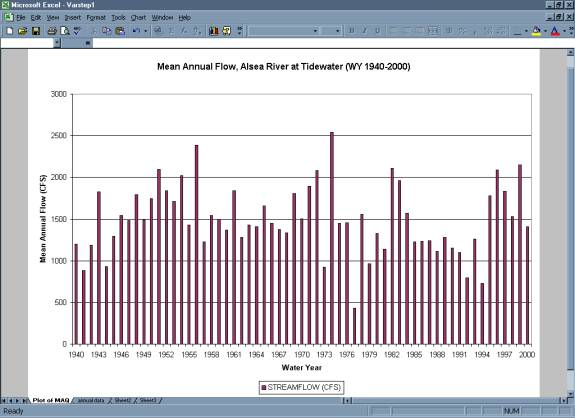• Set the Y-axis to intersect the X-axis at the value for the mean flow for the period of record.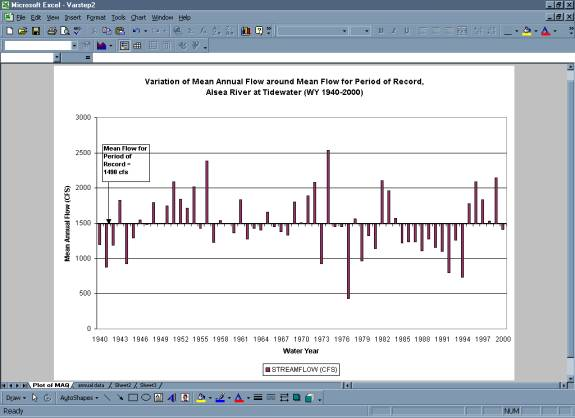### Step 4b:  5-Year and 11-Year Moving Mean

• Starting with the 3rd and 6th year of the period of record, use the average function in Excel to calculate the mean annual flow of the surrounding 5 (yr 1 to yr 5) and 11 (yr 1 to yr 11) years.  Copy these formulas down the columns to calculate the mean annual flow for 5-year and 11-year intervals for the entire period of record. Note that there will be no entry for the last two years in the record for the 5-year moving mean and no entry for the last five years in the record for the 11-year moving mean.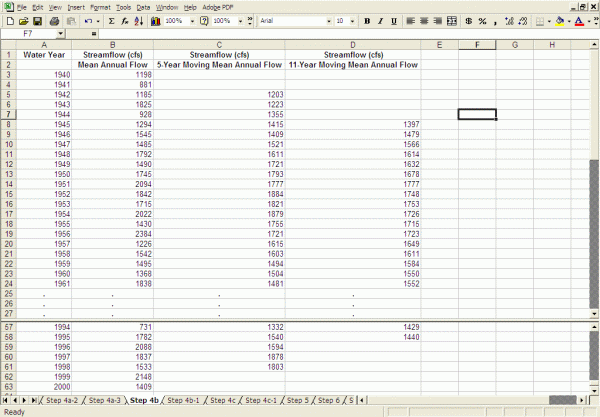• Plot the Average mean annual flow, the 5-year moving mean annual flow, and the 11-year moving mean annual flow.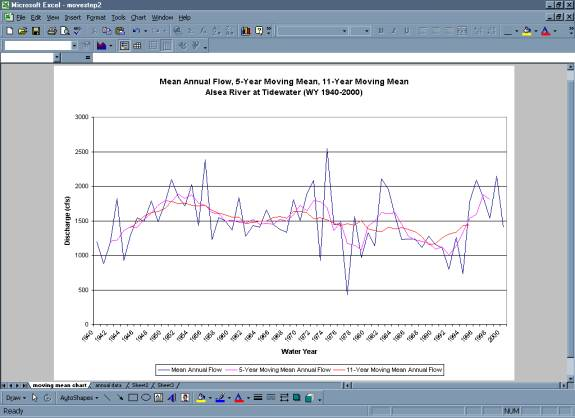### Step 4c:  Mass Curve Method

• Calculate cumulative quantity of streamflow for the period of record using average monthly flows.  See the Data Manipulation section for a more detailed explanation of how to calculate average monthly flows.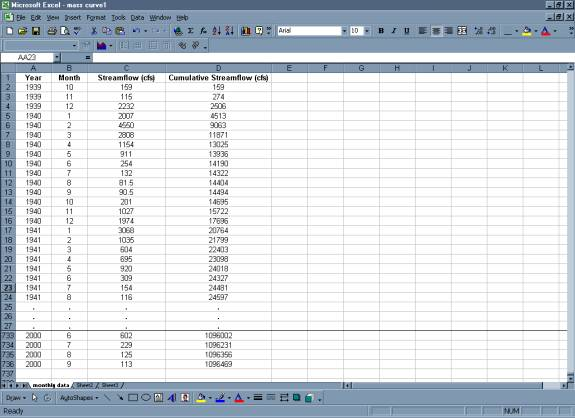• Plot cumulative streamflow versus time.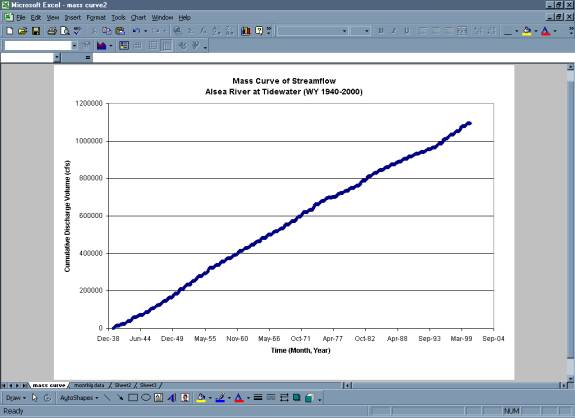### Step 5:  Calculate Simple Statistics

• Excel functions can be used to perform these calculations.
• Mean -- use the AVERAGE function.
• Standard Deviation -- use the STDEV function.
• Maximum Value -- use the MAX function.
• Minimum Value -- use the MIN function.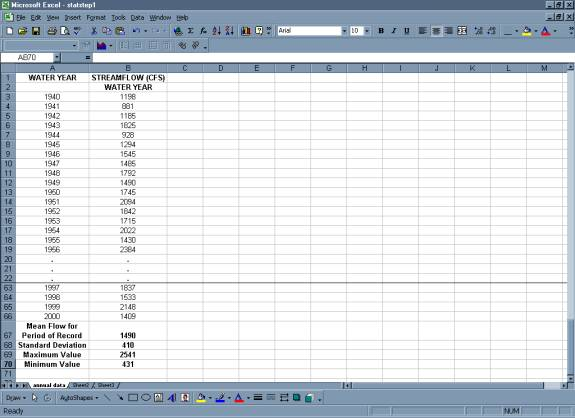### Step 6:  Normalization of Mean Annual Flow (Discharge per Unit Area)

• Calculate Discharge Per Unit Area.
• To do this, you need the Station Description provided by the USGS web page. It includes the drainage area for the gage.

### Show Me (this will open in a separate browser window)

• Mean flow for the Period of Record is divided by the Drainage Area for the gage.